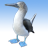cancel
Showing results for
Did you mean:

PSoC™ 5, 3 & 1New Contributor II

ECC Interrupts

} // end of main()

In military and medical applications, it is vital to know if the code you are running is what was written.  That is why the ECC idea is great.  I love that the processor takes care of this issue instead of me having to run a CRC on the Flash at run-time.

I have written a simple piece of code that re-writes an instruction in flash after programming to force a fault.  For example, I set up a simple sum in normal code and then switch it to a subtraction afterwards.  When I turn on ECC mode, the ECC prevents the switch from occurring (great...ECC works!).  I haven't checked, but I imagine the subtract opcode is only 1-bit different from the add opcode.  The trouble is I can't figure out how to register the ISR code when a supposed ECC event occurs.  I have found allusions to this ability in the PSoC 5 datasheet and in several appnotes, but there are no examples or further information.  Does anyone have this working or have any suggestions?  My code is shown below.  It is a variation on what I found in an application note.  I have commented out my attempts at registering the ISR.  I tried a system-level interrupt (only available on the ARM) and a regular one.

#include
volatile uint8 a = 5; // don't let the compiler optimize this away
volatile uint8 b = 3; // don't let the compiler optimize this away
volatile uint8 sum; // don't let the compiler optimize this away

/* To find Row number by dividing the address by 256 */

/* To find address of next row */
#define ROW_INCREMENT SIZEOF_FLASH_ROW

/* Define PSoC 5 Flash Base */
#define FLASH_BASE CYDEV_FLASH_BASE

/* define the ECC interrupt code */
/*
CY_ISR(ECC_Fault_ISR)
{
LCD_ClearDisplay();
LCD_Position(0,0);
LCD_PrintString("PROG MEM FAULT");
}
*/
void main()
{
/* Array which contains value to be written to the Flash Row */
uint8 rowFinalData[SIZEOF_FLASH_ROW];

/* Temp Buffer for Flash line of code*/
uint8 rowBuffer[SIZEOF_FLASH_ROW SIZEOF_ECC_ROW];

/* Start and end locaton of the concerned data */
uint8 rowDataOffset;
uint16 rowDataEnd;

/* Row address for instruction in flash */

uint16 index1;

uint8 runTwice = 0;

// Various attempts at assigning the ECC ISR to the vector table
//CyIntSetSysVector(1, ECC_Fault_ISR);
//CyIntSetVector(1, ECC_Fault_ISR);
//CyIntSetPriority(1, 3);
//CyIntEnable(1);

/* Start LCD */
LCD_Start();

/* To display demo started */
LCD_Position(0,0);
LCD_PrintString("Flash Mem CRC TST");

LCD_Position(1,0);
LCD_PrintNumber(a);
LCD_PrintString(" ");
LCD_PrintNumber(b);
LCD_PrintString("=");

//CYGlobalIntEnable; /* enable global interrupts */

/* create a label for the difference operation. In GCC, this will also be
the address of the operation. This code is never called after the initial
declaration. The instruction will be copied into the SUM flash location after
to simulate a program memory fault. */
DIFOPERATION:
sum = a - b;

while( runTwice != 2 )
{

/* create a label for the difference operation. In GCC, this will also be
the address of the operation. This instruction will get clobbered in flash
as this code executes. I do not recommend re-writing flash too much as this
will weaken the holding-power of the flash. If you really want to test the
program memory fault code every time, then you will need a seperate label
to copy the code back to it's original state. */
SUMOPERATION:
sum = a b;

LCD_Position(1,0);
LCD_PrintNumber(a);
LCD_PrintString(" ");
LCD_PrintNumber(b);
LCD_PrintString("=");

LCD_PrintNumber(sum);
LCD_PrintString(" a:"); // show address for verification
LCD_PrintHexUint16( &&SUMOPERATION );
LCD_PrintString(" ");
LCD_PrintNumber(runTwice);

CyDelay(2000); // wait 2 seconds

if( runTwice == 0 )
{
/* Get the address where the sine lookup table is stored */

/* Calculate the row number from the address, which is the remainder of address/256 (max row size) */

/* Calculate the starting address of row */

for(index1 = 0; index1 < SIZEOF_FLASH_ROW; index1 )
{
if ( index1 == rowDataOffset )
{
rowFinalData[index1] = (uint16) (void*)(&&DIFOPERATION); // replace sum operation with difference operation
}
else
{
/* Save the other elements of the flash row in the same position as they were */
rowFinalData[index1] = CY_GET_XTND_REG8((const void *)(FLASH_BASE rowAddress1 index1));
}
}

/* Must get temperature and set a temp buffer first. My understanding is different power is used
to write to the flash depending upon its temperature. */
if(!CySetTemp() && !CySetFlashEEBuffer(rowBuffer))
{
/* Erase and Program the row. */
}
runTwice = 1;
}
else
{
runTwice = 2;
}
}
while( 1 )
{
/* Never exit */
}